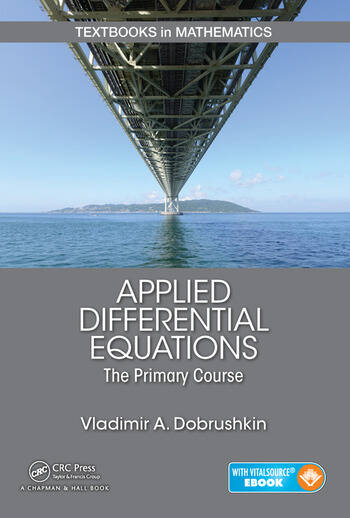# Applied Differential Equations: The Primary Course

## 1st Edition

Chapman and Hall/CRC
Published December 16, 2014
Textbook - 731 Pages - 191 B/W Illustrations
ISBN 9781439851043 - CAT# K12330
Series: Textbooks in Mathematics

For Instructors Request Inspection Copy

was \$125.00

USD\$100.00

SAVE ~\$25.00

FREE Standard Shipping!

## Preview

### Summary

A Contemporary Approach to Teaching Differential Equations

Applied Differential Equations: An Introduction presents a contemporary treatment of ordinary differential equations (ODEs) and an introduction to partial differential equations (PDEs), including their applications in engineering and the sciences. Designed for a two-semester undergraduate course, the text offers a true alternative to books published for past generations of students. It enables students majoring in a range of fields to obtain a solid foundation in differential equations.

The text covers traditional material, along with novel approaches to mathematical modeling that harness the capabilities of numerical algorithms and popular computer software packages. It contains practical techniques for solving the equations as well as corresponding codes for numerical solvers. Many examples and exercises help students master effective solution techniques, including reliable numerical approximations.

This book describes differential equations in the context of applications and presents the main techniques needed for modeling and systems analysis. It teaches students how to formulate a mathematical model, solve differential equations analytically and numerically, analyze them qualitatively, and interpret the results.

#### Instructors

We provide complimentary e-inspection copies of primary textbooks to instructors considering our books for course adoption.